# FADML 06 PPC Graphs and Traversals.pdf

1 de Apr de 2023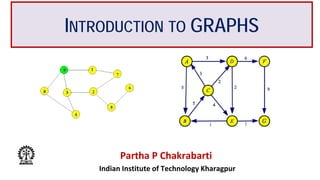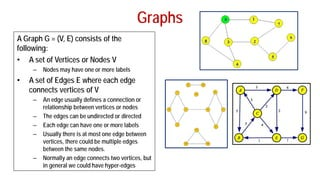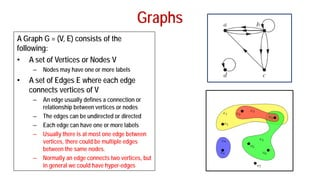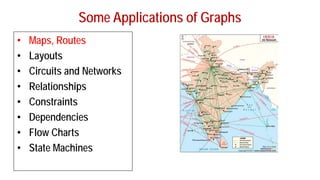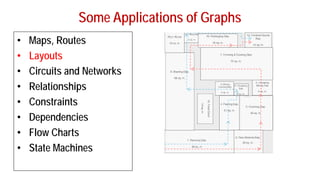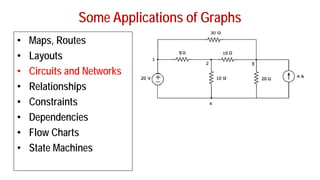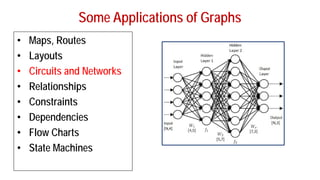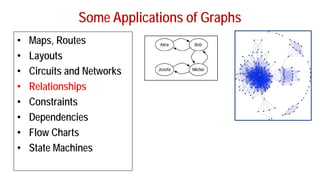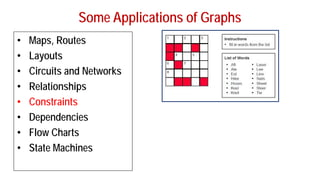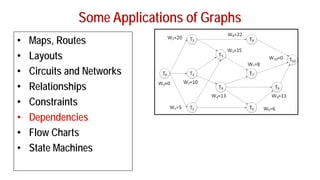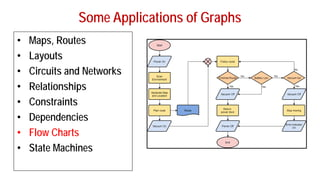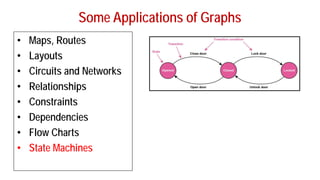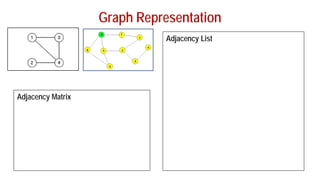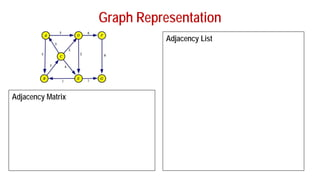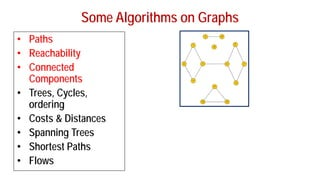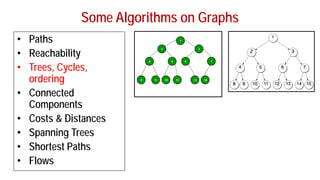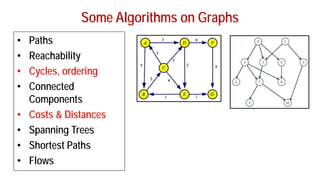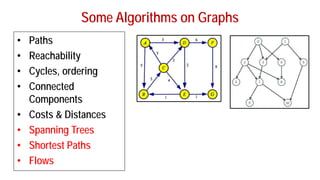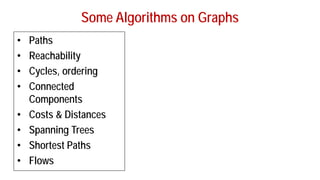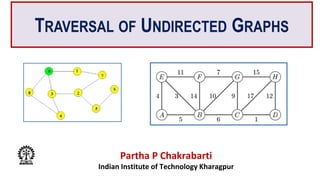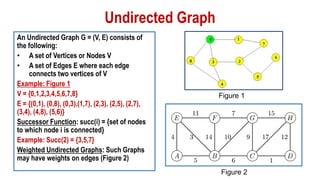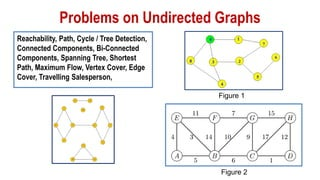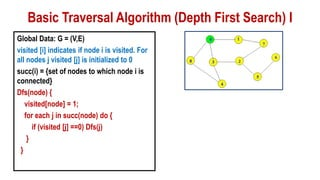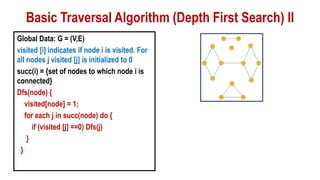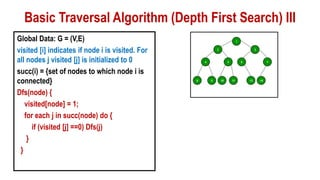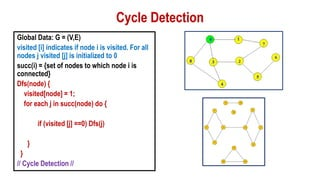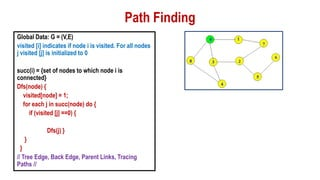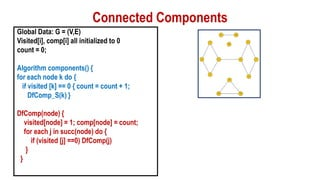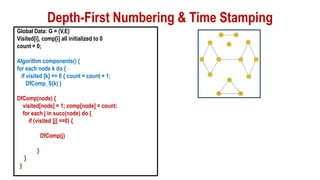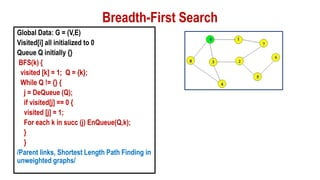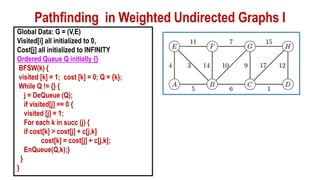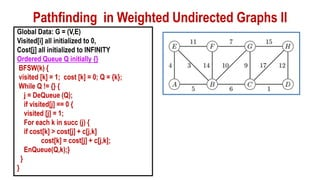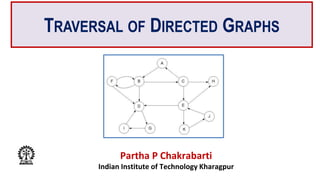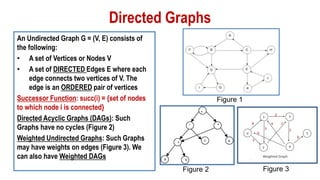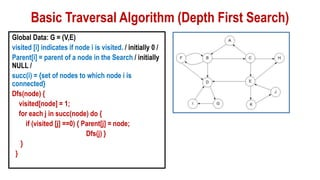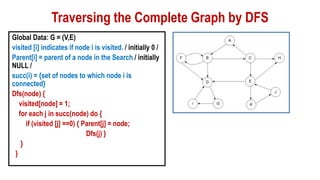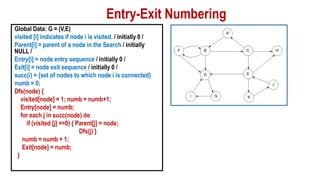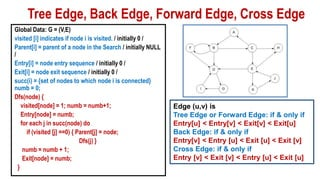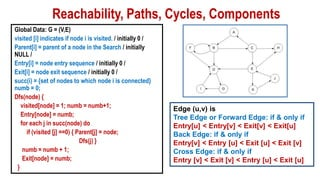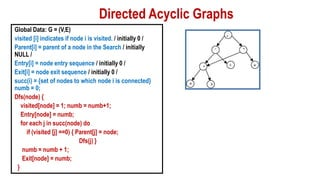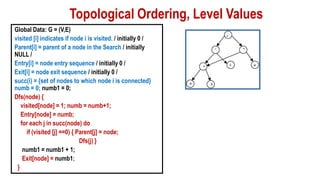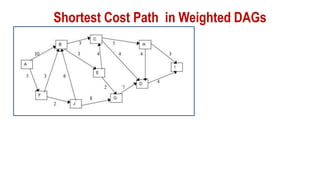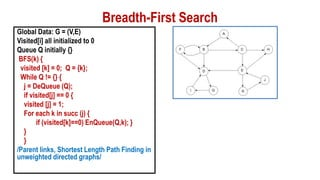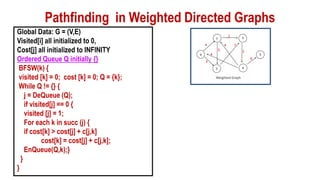1 de 47

### FADML 06 PPC Graphs and Traversals.pdf

1. INTRODUCTION TO GRAPHS Partha P Chakrabarti Indian Institute of Technology Kharagpur
2. Graphs A Graph G = (V, E) consists of the following: • A set of Vertices or Nodes V – Nodes may have one or more labels • A set of Edges E where each edge connects vertices of V – An edge usually defines a connection or relationship between vertices or nodes – The edges can be undirected or directed – Each edge can have one or more labels – Usually there is at most one edge between vertices, there could be multiple edges between the same nodes. – Normally an edge connects two vertices, but in general we could have hyper-edges
3. Graphs A Graph G = (V, E) consists of the following: • A set of Vertices or Nodes V – Nodes may have one or more labels • A set of Edges E where each edge connects vertices of V – An edge usually defines a connection or relationship between vertices or nodes – The edges can be undirected or directed – Each edge can have one or more labels – Usually there is at most one edge between vertices, there could be multiple edges between the same nodes. – Normally an edge connects two vertices, but in general we could have hyper-edges
4. Some Applications of Graphs • Maps, Routes • Layouts • Circuits and Networks • Relationships • Constraints • Dependencies • Flow Charts • State Machines
5. Some Applications of Graphs • Maps, Routes • Layouts • Circuits and Networks • Relationships • Constraints • Dependencies • Flow Charts • State Machines
6. Some Applications of Graphs • Maps, Routes • Layouts • Circuits and Networks • Relationships • Constraints • Dependencies • Flow Charts • State Machines
7. Some Applications of Graphs • Maps, Routes • Layouts • Circuits and Networks • Relationships • Constraints • Dependencies • Flow Charts • State Machines
8. Some Applications of Graphs • Maps, Routes • Layouts • Circuits and Networks • Relationships • Constraints • Dependencies • Flow Charts • State Machines
9. Some Applications of Graphs • Maps, Routes • Layouts • Circuits and Networks • Relationships • Constraints • Dependencies • Flow Charts • State Machines
10. Some Applications of Graphs • Maps, Routes • Layouts • Circuits and Networks • Relationships • Constraints • Dependencies • Flow Charts • State Machines
11. Some Applications of Graphs • Maps, Routes • Layouts • Circuits and Networks • Relationships • Constraints • Dependencies • Flow Charts • State Machines
12. Some Applications of Graphs • Maps, Routes • Layouts • Circuits and Networks • Relationships • Constraints • Dependencies • Flow Charts • State Machines
15. Some Algorithms on Graphs • Paths • Reachability • Connected Components • Trees, Cycles, ordering • Costs & Distances • Spanning Trees • Shortest Paths • Flows
16. Some Algorithms on Graphs • Paths • Reachability • Trees, Cycles, ordering • Connected Components • Costs & Distances • Spanning Trees • Shortest Paths • Flows
17. Some Algorithms on Graphs • Paths • Reachability • Cycles, ordering • Connected Components • Costs & Distances • Spanning Trees • Shortest Paths • Flows
18. Some Algorithms on Graphs • Paths • Reachability • Cycles, ordering • Connected Components • Costs & Distances • Spanning Trees • Shortest Paths • Flows
19. Some Algorithms on Graphs • Paths • Reachability • Cycles, ordering • Connected Components • Costs & Distances • Spanning Trees • Shortest Paths • Flows
20. Thank you
21. TRAVERSAL OF UNDIRECTED GRAPHS Partha P Chakrabarti Indian Institute of Technology Kharagpur
22. Undirected Graph An Undirected Graph G = (V, E) consists of the following: • A set of Vertices or Nodes V • A set of Edges E where each edge connects two vertices of V Example: Figure 1 V = {0,1,2,3,4,5,6,7,8} E = {(0,1), (0,8), (0,3),(1,7), (2,3), (2,5), (2,7), (3,4), (4,8), (5,6)} Successor Function: succ(i) = {set of nodes to which node i is connected} Example: Succ(2) = {3,5,7} Weighted Undirected Graphs: Such Graphs may have weights on edges (Figure 2) Figure 1 Figure 2
23. Problems on Undirected Graphs Reachability, Path, Cycle / Tree Detection, Connected Components, Bi-Connected Components, Spanning Tree, Shortest Path, Maximum Flow, Vertex Cover, Edge Cover, Travelling Salesperson, Figure 1 Figure 2
24. Basic Traversal Algorithm (Depth First Search) I Global Data: G = (V,E) visited [i] indicates if node i is visited. For all nodes j visited [j] is initialized to 0 succ(i) = {set of nodes to which node i is connected} Dfs(node) { visited[node] = 1; for each j in succ(node) do { if (visited [j] ==0) Dfs(j) } }
25. Basic Traversal Algorithm (Depth First Search) II Global Data: G = (V,E) visited [i] indicates if node i is visited. For all nodes j visited [j] is initialized to 0 succ(i) = {set of nodes to which node i is connected} Dfs(node) { visited[node] = 1; for each j in succ(node) do { if (visited [j] ==0) Dfs(j) } }
26. Basic Traversal Algorithm (Depth First Search) III Global Data: G = (V,E) visited [i] indicates if node i is visited. For all nodes j visited [j] is initialized to 0 succ(i) = {set of nodes to which node i is connected} Dfs(node) { visited[node] = 1; for each j in succ(node) do { if (visited [j] ==0) Dfs(j) } }
27. Cycle Detection Global Data: G = (V,E) visited [i] indicates if node i is visited. For all nodes j visited [j] is initialized to 0 succ(i) = {set of nodes to which node i is connected} Dfs(node) { visited[node] = 1; for each j in succ(node) do { if (visited [j] ==0) Dfs(j) } } // Cycle Detection //
28. Path Finding Global Data: G = (V,E) visited [i] indicates if node i is visited. For all nodes j visited [j] is initialized to 0 succ(i) = {set of nodes to which node i is connected} Dfs(node) { visited[node] = 1; for each j in succ(node) do { if (visited [j] ==0) { Dfs(j) } } } // Tree Edge, Back Edge, Parent Links, Tracing Paths //
29. Connected Components Global Data: G = (V,E) Visited[i], comp[i] all initialized to 0 count = 0; Algorithm components() { for each node k do { if visited [k] == 0 { count = count + 1; DfComp_S(k) } DfComp(node) { visited[node] = 1; comp[node] = count; for each j in succ(node) do { if (visited [j] ==0) DfComp(j) } }
30. Depth-First Numbering & Time Stamping Global Data: G = (V,E) Visited[i], comp[i] all initialized to 0 count = 0; Algorithm components() { for each node k do { if visited [k] == 0 { count = count + 1; DfComp_S(k) } DfComp(node) { visited[node] = 1; comp[node] = count; for each j in succ(node) do { if (visited [j] ==0) { DfComp(j) } } }
31. Breadth-First Search Global Data: G = (V,E) Visited[i] all initialized to 0 Queue Q initially {} BFS(k) { visited [k] = 1; Q = {k}; While Q != {} { j = DeQueue (Q); if visited[j] == 0 { visited [j] = 1; For each k in succ (j) EnQueue(Q,k); } } /Parent links, Shortest Length Path Finding in unweighted graphs/
32. Pathfinding in Weighted Undirected Graphs I Global Data: G = (V,E) Visited[i] all initialized to 0, Cost[j] all initialized to INFINITY Ordered Queue Q initially {} BFSW(k) { visited [k] = 1; cost [k] = 0; Q = {k}; While Q != {} { j = DeQueue (Q); if visited[j] == 0 { visited [j] = 1; For each k in succ (j) { if cost[k] > cost[j] + c[j,k] cost[k] = cost[j] + c[j,k]; EnQueue(Q,k);} } }
33. Pathfinding in Weighted Undirected Graphs II Global Data: G = (V,E) Visited[i] all initialized to 0, Cost[j] all initialized to INFINITY Ordered Queue Q initially {} BFSW(k) { visited [k] = 1; cost [k] = 0; Q = {k}; While Q != {} { j = DeQueue (Q); if visited[j] == 0 { visited [j] = 1; For each k in succ (j) { if cost[k] > cost[j] + c[j,k] cost[k] = cost[j] + c[j,k]; EnQueue(Q,k);} } }
34. Thank you
35. TRAVERSAL OF DIRECTED GRAPHS Partha P Chakrabarti Indian Institute of Technology Kharagpur
36. Directed Graphs An Undirected Graph G = (V, E) consists of the following: • A set of Vertices or Nodes V • A set of DIRECTED Edges E where each edge connects two vertices of V. The edge is an ORDERED pair of vertices Successor Function: succ(i) = {set of nodes to which node i is connected} Directed Acyclic Graphs (DAGs): Such Graphs have no cycles (Figure 2) Weighted Undirected Graphs: Such Graphs may have weights on edges (Figure 3). We can also have Weighted DAGs Figure 1 Figure 3 Figure 2
37. Basic Traversal Algorithm (Depth First Search) Global Data: G = (V,E) visited [i] indicates if node i is visited. / initially 0 / Parent[i] = parent of a node in the Search / initially NULL / succ(i) = {set of nodes to which node i is connected} Dfs(node) { visited[node] = 1; for each j in succ(node) do { if (visited [j] ==0) { Parent[j] = node; Dfs(j) } } }
38. Traversing the Complete Graph by DFS Global Data: G = (V,E) visited [i] indicates if node i is visited. / initially 0 / Parent[i] = parent of a node in the Search / initially NULL / succ(i) = {set of nodes to which node i is connected} Dfs(node) { visited[node] = 1; for each j in succ(node) do { if (visited [j] ==0) { Parent[j] = node; Dfs(j) } } }
39. Global Data: G = (V,E) visited [i] indicates if node i is visited. / initially 0 / Parent[i] = parent of a node in the Search / initially NULL / Entry[i] = node entry sequence / initially 0 / Exit[i] = node exit sequence / initially 0 / succ(i) = {set of nodes to which node i is connected} numb = 0; Dfs(node) { visited[node] = 1; numb = numb+1; Entry[node] = numb; for each j in succ(node) do if (visited [j] ==0) { Parent[j] = node; Dfs(j) } numb = numb + 1; Exit[node] = numb; } Entry-Exit Numbering
40. Global Data: G = (V,E) visited [i] indicates if node i is visited. / initially 0 / Parent[i] = parent of a node in the Search / initially NULL / Entry[i] = node entry sequence / initially 0 / Exit[i] = node exit sequence / initially 0 / succ(i) = {set of nodes to which node i is connected} numb = 0; Dfs(node) { visited[node] = 1; numb = numb+1; Entry[node] = numb; for each j in succ(node) do if (visited [j] ==0) { Parent[j] = node; Dfs(j) } numb = numb + 1; Exit[node] = numb; } Tree Edge, Back Edge, Forward Edge, Cross Edge Edge (u,v) is Tree Edge or Forward Edge: if & only if Entry[u] < Entry[v] < Exit[v] < Exit[u] Back Edge: if & only if Entry[v] < Entry [u] < Exit [u] < Exit [v] Cross Edge: if & only if Entry [v] < Exit [v] < Entry [u] < Exit [u]
41. Global Data: G = (V,E) visited [i] indicates if node i is visited. / initially 0 / Parent[i] = parent of a node in the Search / initially NULL / Entry[i] = node entry sequence / initially 0 / Exit[i] = node exit sequence / initially 0 / succ(i) = {set of nodes to which node i is connected} numb = 0; Dfs(node) { visited[node] = 1; numb = numb+1; Entry[node] = numb; for each j in succ(node) do if (visited [j] ==0) { Parent[j] = node; Dfs(j) } numb = numb + 1; Exit[node] = numb; } Reachability, Paths, Cycles, Components Edge (u,v) is Tree Edge or Forward Edge: if & only if Entry[u] < Entry[v] < Exit[v] < Exit[u] Back Edge: if & only if Entry[v] < Entry [u] < Exit [u] < Exit [v] Cross Edge: if & only if Entry [v] < Exit [v] < Entry [u] < Exit [u]
42. Global Data: G = (V,E) visited [i] indicates if node i is visited. / initially 0 / Parent[i] = parent of a node in the Search / initially NULL / Entry[i] = node entry sequence / initially 0 / Exit[i] = node exit sequence / initially 0 / succ(i) = {set of nodes to which node i is connected} numb = 0; Dfs(node) { visited[node] = 1; numb = numb+1; Entry[node] = numb; for each j in succ(node) do if (visited [j] ==0) { Parent[j] = node; Dfs(j) } numb = numb + 1; Exit[node] = numb; } Directed Acyclic Graphs
43. Global Data: G = (V,E) visited [i] indicates if node i is visited. / initially 0 / Parent[i] = parent of a node in the Search / initially NULL / Entry[i] = node entry sequence / initially 0 / Exit[i] = node exit sequence / initially 0 / succ(i) = {set of nodes to which node i is connected} numb = 0; numb1 = 0; Dfs(node) { visited[node] = 1; numb = numb+1; Entry[node] = numb; for each j in succ(node) do if (visited [j] ==0) { Parent[j] = node; Dfs(j) } numb1 = numb1 + 1; Exit[node] = numb1; } Topological Ordering, Level Values
44. Shortest Cost Path in Weighted DAGs
45. Breadth-First Search Global Data: G = (V,E) Visited[i] all initialized to 0 Queue Q initially {} BFS(k) { visited [k] = 0; Q = {k}; While Q != {} { j = DeQueue (Q); if visited[j] == 0 { visited [j] = 1; For each k in succ (j) { if (visited[k]==0) EnQueue(Q,k); } } } /Parent links, Shortest Length Path Finding in unweighted directed graphs/
46. Pathfinding in Weighted Directed Graphs Global Data: G = (V,E) Visited[i] all initialized to 0, Cost[j] all initialized to INFINITY Ordered Queue Q initially {} BFSW(k) { visited [k] = 0; cost [k] = 0; Q = {k}; While Q != {} { j = DeQueue (Q); if visited[j] == 0 { visited [j] = 1; For each k in succ (j) { if cost[k] > cost[j] + c[j,k] cost[k] = cost[j] + c[j,k]; EnQueue(Q,k);} } }
47. Thank you